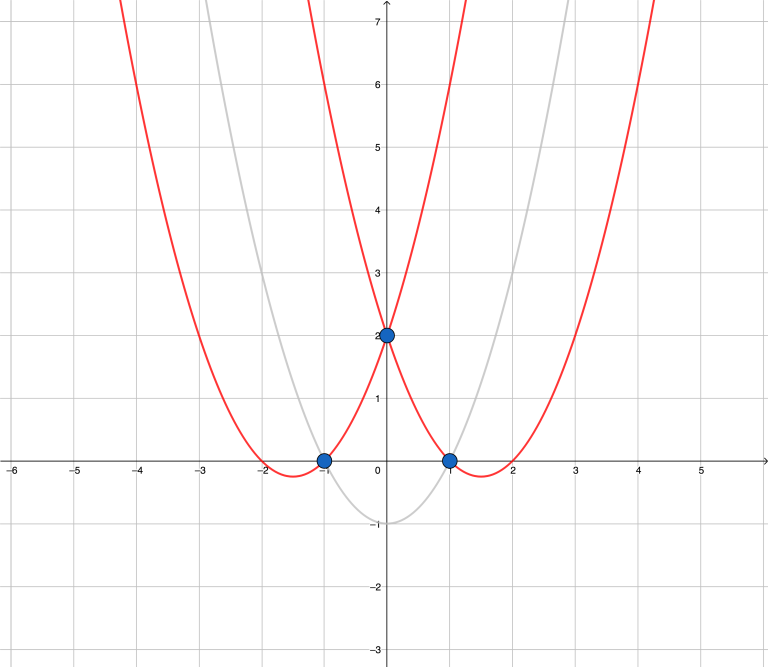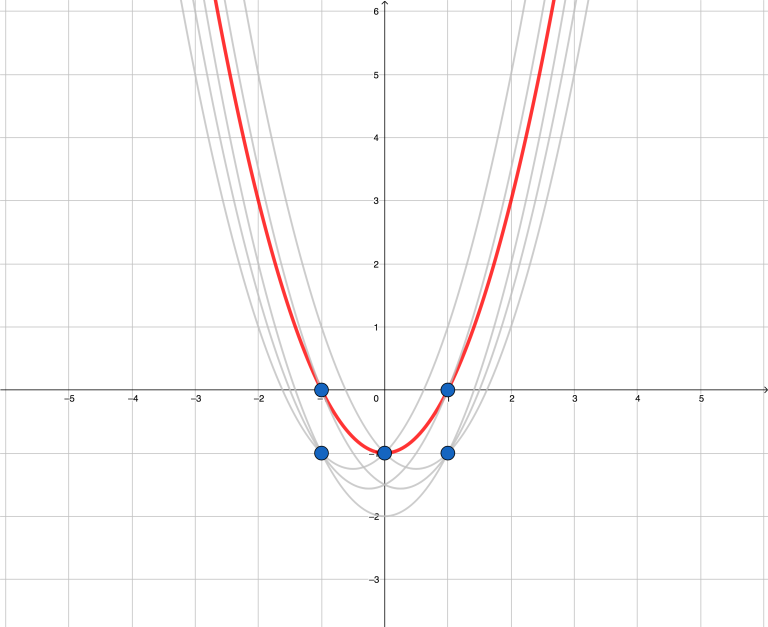# CF1142C U2

• 33通过
• 86提交
• 题目来源
• 评测方式 RemoteJudge
• 标签
• 难度 省选/NOI-
• 时空限制 1000ms / 256MB
• 提示：收藏到任务计划后，可在首页查看。

## 题意翻译

有 $n$ 个互不相同的点 $(x_i,y_i)$ ，对于所有无序二元组 $(i,j)$ （满足 $x_i\neq x_j$ ），过点 $i$ 和点 $j$ 作一条解析式为 $y=x^2+bx+c$ 的抛物线。求有多少条作出的抛物线（重合的算作一条）满足其（严格）上方的区域没有任何一个点。

## 题目描述

Recently Vasya learned that, given two points with different $x$ coordinates, you can draw through them exactly one parabola with equation of type $y = x^2 + bx + c$ , where $b$ and $c$ are reals. Let's call such a parabola an $U$ -shaped one.

Vasya drew several distinct points with integer coordinates on a plane and then drew an $U$ -shaped parabola through each pair of the points that have different $x$ coordinates. The picture became somewhat messy, but Vasya still wants to count how many of the parabolas drawn don't have any drawn point inside their internal area. Help Vasya.

The internal area of an $U$ -shaped parabola is the part of the plane that lies strictly above the parabola when the $y$ axis is directed upwards.

## 输入输出格式

输入格式：

The first line contains a single integer $n$ ( $1 \le n \le 100\,000$ ) — the number of points.

The next $n$ lines describe the points, the $i$ -th of them contains two integers $x_i$ and $y_i$ — the coordinates of the $i$ -th point. It is guaranteed that all points are distinct and that the coordinates do not exceed $10^6$ by absolute value.

输出格式：

In the only line print a single integer — the number of $U$ -shaped parabolas that pass through at least two of the given points and do not contain any of the given points inside their internal area (excluding the parabola itself).

## 输入输出样例

输入样例#1： 复制
3
-1 0
0 2
1 0

输出样例#1： 复制
2

输入样例#2： 复制
5
1 0
1 -1
0 -1
-1 0
-1 -1

输出样例#2： 复制
1


## 说明

On the pictures below all $U$ -shaped parabolas that pass through at least two given points are drawn for each of the examples. The $U$ -shaped parabolas that do not have any given point inside their internal area are drawn in red.The first example.The second example.

提示
标程仅供做题后或实在无思路时参考。
请自觉、自律地使用该功能并请对自己的学习负责。
如果发现恶意抄袭标程，将按照I类违反进行处理。